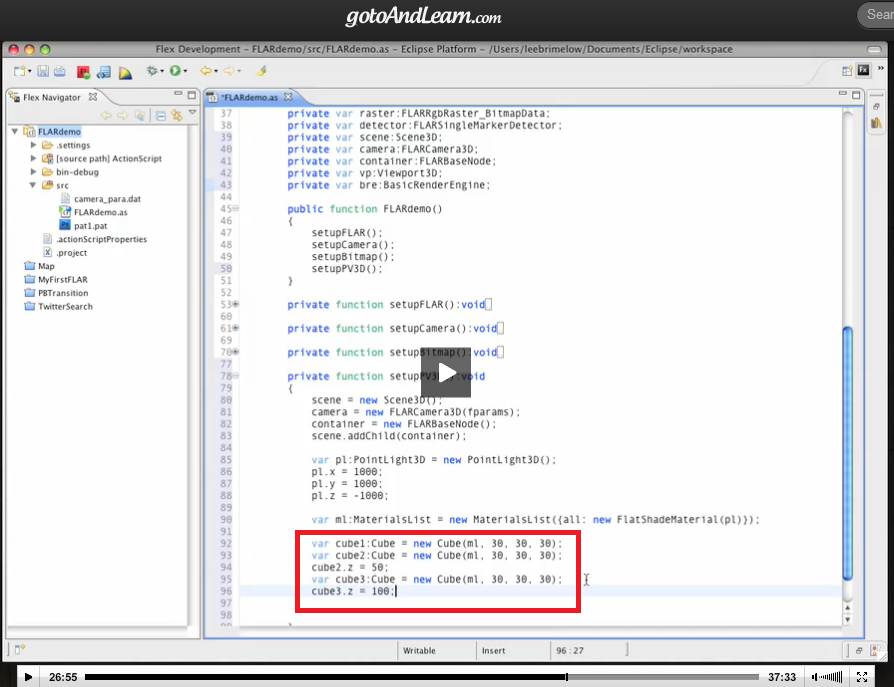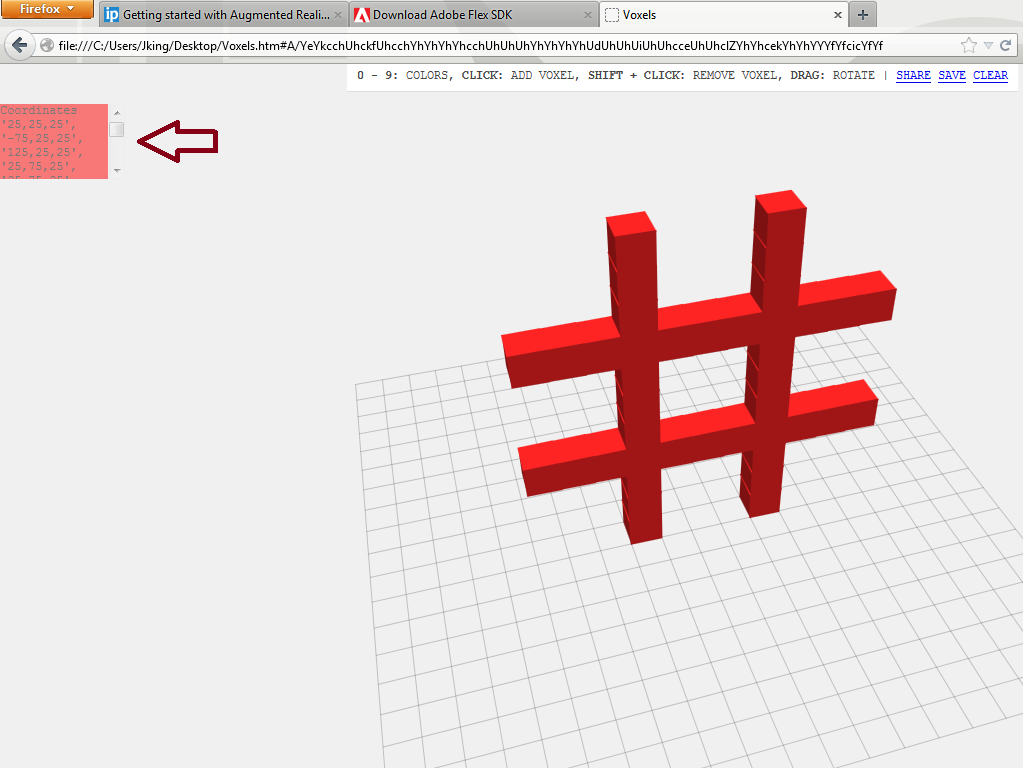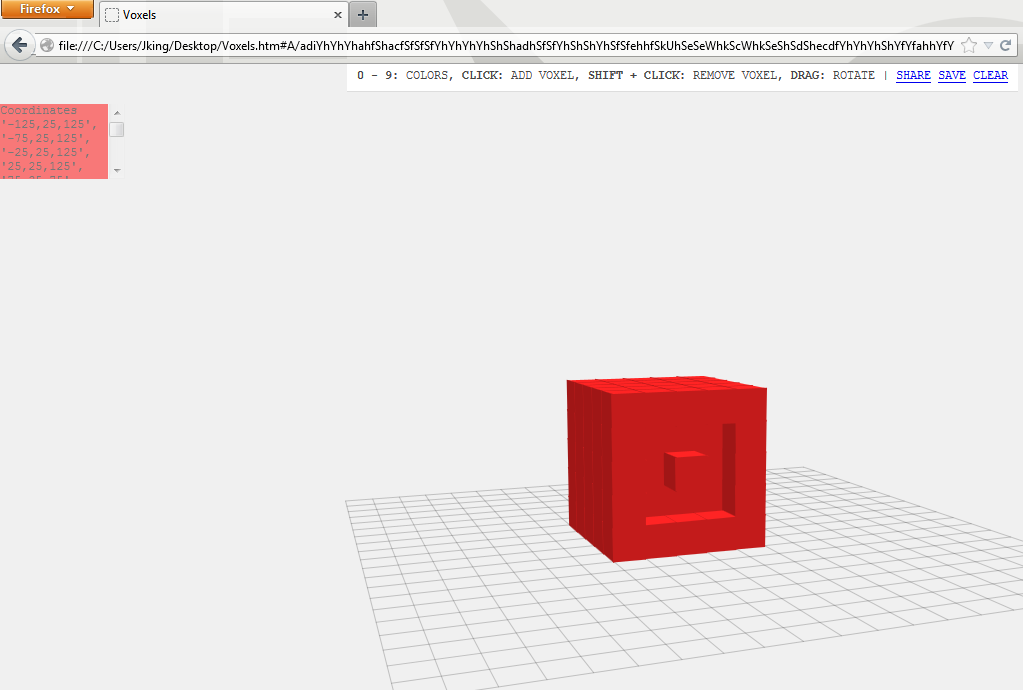# Voxels as a Makeshift 3D Editor

Original Date: August 2, 2013

While following this tutorial from Lee Brimelow, I noticed that he made the shapes using cubes.Which reminded me of the Voxels program by Mr.doob I had stumbled upon earlier. I figured, hey I could use this!

I downloaded a copy and added some lines to the source code so that it would show the coordinates of the cubes.

And it worked out!All the lovely details

1) Added the following lines to display the coordinates

``````
var coordinates = document.createElement( 'div');
coordinates.style.backgroundColor = '#FF0000';
coordinates.style.cssFloat = 'right';
coordinates.style.marginTop = '40px';
coordinates.style.position = 'absolute';
coordinates.style.overflow = 'auto';
coordinates.style.height = '75px';
coordinates.style.width = '125px';
coordinates.style.opacity = '0.5';
``````

2) This was my workaround to track which cubes were omitted

``````
// To note addition of a cube
var santa = document.getElementById("coordinates");
var newline = document.createElement('div');
newline.innerHTML = ( "'" + voxel.position.x + "," + voxel.position.y + "," + voxel.position.z + "', ");
while (newline.firstChild) {
santa.appendChild(newline.firstChild);
}
//http://stackoverflow.com/questions/595808/is-it-possible-to-append-to-innerhtml-without-destroying-descendants-onclick-fu
``````
``````
//To note omission of a cube
var santa2 = document.getElementById("coordinates");
var newline2 = document.createElement('div');
newline2.innerHTML = ("'" + intersect.object.position.x + "," + intersect.object.position.y + "," + intersect.object.position.z + "', ");
while (newline2.firstChild) {
santa2.appendChild(newline2.firstChild);
}
``````

And in a separate file

``````
//Script designed to handle voxel cubes that are erased
/*Logic:
The coordinates logged during the addition/omission of a cube are the same. The sequence is add/omit/add/omit etc.
Thus if a coordinate appears an odd number of times, the end goal is for it to be added. Ditto for even, where the endgoal is omission
*/

//Count occurrences of each element in the array
//Code by Sime Vdas (http://stackoverflow.com/a/5668246)
function foo(arr) {
var a = [], b = [], prev;

arr.sort();
for ( var i = 0; i < arr.length; i++ ) {
if ( arr[i] !== prev ) {
a.push(arr[i]);
b.push(1);
} else {
b[b.length-1]++;
}
prev = arr[i];
}

return [a, b];
}
var result = foo(arr);

//Create new arrays from result
var values = result;         //output of all unique values
var instances = result;      //number of instances for each value

//Remove elements that occur an even number of times
for ( var i = 0; i < instances.length; i++ ) {
if (instances[i] %2 === 0){
values.splice(i,1);
instances.splice(i,1);
};
}

//Display the trimmed array
document.write (values.join("")) //write each element in its own line
``````

▱ Voxels tweaked to show coordinates

Update (April 2015):

If I was to rewrite this code today… there would be no need for all that code! Or more importantly, a second file!

Specifically, I would just append each cube’s x,y,z positions to an array. As such,

``````
myArray = [[x,y,z], [x,y,z], [x,y,z]];
``````

Include the odd/even element count logic above as a function,

``````
function removeOmitted( array ){
//odd even count logic modified to return an array
}
``````

Call it on the array,

``````
myFinalArray = removeOmitted( myArray );
``````

And then display these coordinates

``````
var santa = document.getElementById("coordinates");
for(var i = 0; i < myFinalArray.length; i++){
santa.innerHTML += myFinalArray[i] + ", " + myFinalArray[i] + ", " + myFinalArray[i] + "";
}
``````

At the time I did not know much about arrays or functions. Though I am certain if I revisit my ‘update’ a few years from now I will probably be cringing! =)